The objective of this study is to predict human wine taste preferences.

This can be useful to improve wine production and to support the oenologist’s wine tasting evaluations.

Furthermore, similar techniques can help target marketing by modeling consumer tastes from niche markets.

The variables used for this proposal are not related to grape type, wine brand, or wine selling price; they are only associated with physicochemical tests. The model’s output will give a score between 0 and 10, which defines the wine quality.

### Contents

This example is solved with Neural Designer. To follow it step by step, you can use the free trial.

## 1. Application type

This is an approximation project since the variable to be predicted is continuous (wine quality).

The fundamental goal here is to model the quality of a wine as a function of its features.

## 2. Data set

The data file wine_quality.csv contains a total of 1599 rows and 12 columns. The first row in the data file contains the names of the variables, and the rest of them represent the instances.

The data set contains the following variables:

• fixed_acidity
• volatile_acidity
• citric_acid
• residual_sugar
• chlorides
• free_sulfur_dioxide
• total_sulfur_dioxide
• density
• pH
• sulfates
• alcohol
• quality

On the other hand, the instances are divided randomly into training, selection, and testing subsets, containing 60%, 20%, and 20% of the instances, respectively.

Once the data set page has been edited, we will run a few related tasks. With that, we check that the provided information is of good quality.

We can calculate the data distributions and draw a histogram for each variable to see how they are distributed. The following figure shows the histogram for the quality data, which is the only target.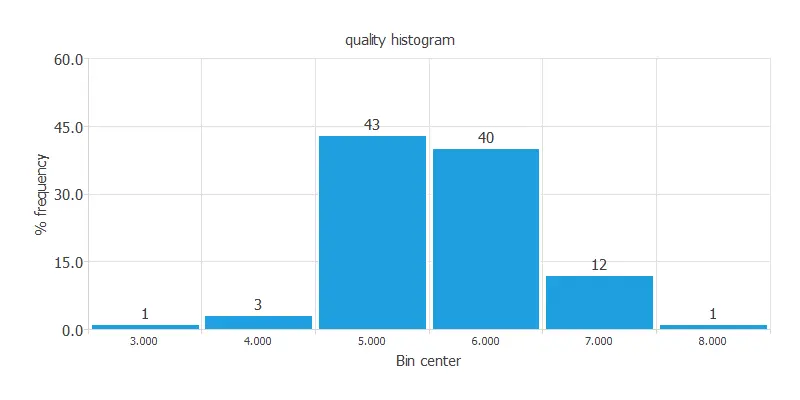As we can see, the target variable is very unbalanced since there are many quality scores around six and much less with values near 0 or 10. This will result in a poor-quality model.

The following chart shows the correlations of the input variables with the target variable.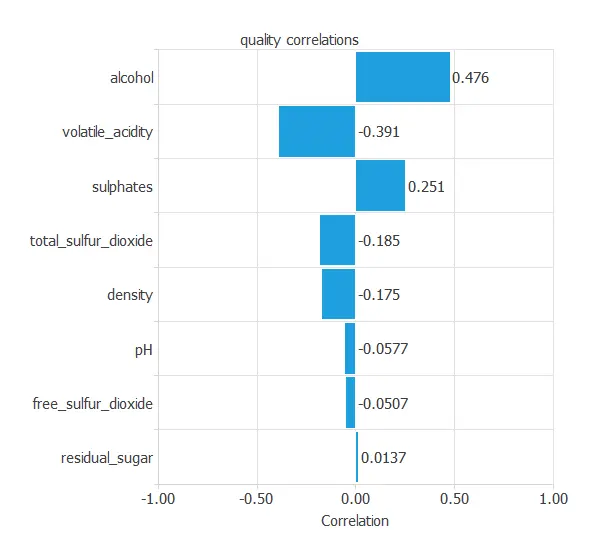### 3. Neural network

The second step is to set the neural network stuff. For approximation, project types, it is usually composed of:

• Scaling layer.
• Perceptron layers.
• Unscaling layer.
• Bounding layer.

The scaling layer section contains the statistics on the inputs calculated from the data file and the method for scaling the input variables. Here the mean and standard deviation scaling method has been set. Nevertheless, the mean and standard deviation methods would produce very similar results.

A hidden layer and a linear output layer of perceptrons will be used in this problem (this is the default in approximation). It must have ten inputs and one output neuron. While the problem constrains inputs and output neurons, the number of neurons in the hidden layer is a design variable. Here we use three neurons in the hidden layer, yielding 37 parameters. Finally, all the biases and synaptic weights in the neural network are initialized randomly.

The unscaling layer contains the statistics on the outputs calculated from the data file and the method for unscaling the output variables. Here the minimum and maximum unscaling method will be used.

At last, in this example, we will use a bounding layer, with 1 and 10 as the lower bound and upper bound, respectively. This is because the quality variable’s value clearly defines lower and upper limits.

The neural network for this example can be represented as the following diagram: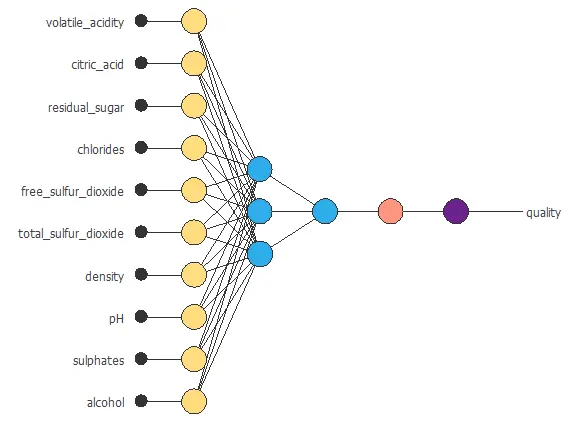The function above is parameterized by all the biases and synaptic weights in the neural network, i.e., 37 parameters.

## 4. Training strategy

The next step is to select an appropriate training strategy, which defines what the neural network will learn. A general training strategy for approximation is composed of two terms:

• A loss index.
• An optimization algorithm.

The loss index chosen for this problem is the normalized squared error between the outputs from the neural network and the targets in the data set. On the other hand, L2 regularization with weak weight is applied here.

The selected optimization algorithm is the quasi-Newton method.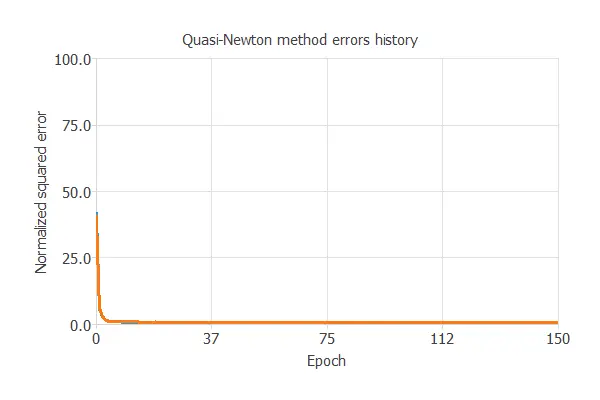The most crucial training result is the final selection error. Indeed, this is a measure of the generalization capabilities of the neural network. Here the final selection error is selection error = 0.678 NSE.

## 5. Model selection

The objective of model selection is to find the network architecture with the best generalization properties, that is, that which minimizes the error on the selected instances of the data set.

More specifically, we want to find a neural network with a selection error of less than 0.678 NSE, which is the value that we have achieved so far.

Order selection algorithms train several network architectures with a different number of neurons and select that with the smallest selection error.

The incremental order method starts with a small number of neurons and increases the complexity at each iteration. The following chart shows the training error (blue) and the selection error (orange) as a function of the number of neurons.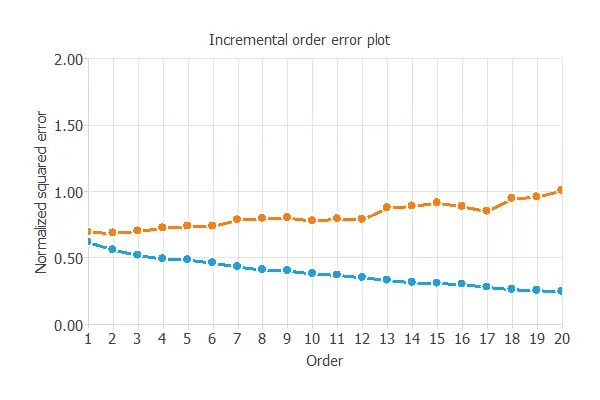The final selection error achieved is 0.661 for an optimal number of neurons of 1.The graph above represents the architecture of the final neural network.

## 6. Testing analysis

A standard method for testing the prediction capabilities is to compare the outputs from the neural network against an independent set of data. The linear regression analysis leads to 3 parameters for each output: intercept, slope, and correlation. The next figure shows the results of this analysis.If the correlation is equal to 1, then there is a perfect correlation between the outputs from the neural network and the targets in the testing subset. For this case, the correlation has an R2 = 0.58, which indicates that the model is not predicting very well.

As stated previously, the quality data is remarkably unbalanced; this can be observed in the previous graph.

## 7. Model deployment

The model obtained after all the steps are not the best it could be achieved. Nevertheless, it is still better than guessing randomly.

The next listing shows the mathematical expression of the predictive model.

scaled_volatile_acidity = (volatile_acidity-0.527821)/0.17906;
scaled_citric_acid = (citric_acid-0.270976)/0.194801;
scaled_residual_sugar = (residual_sugar-2.53881)/1.40993;
scaled_chlorides = (chlorides-0.0874665)/0.0470653;
scaled_free_sulfur_dioxide = (free_sulfur_dioxide-15.8749)/10.4602;
scaled_total_sulfur_dioxide = (total_sulfur_dioxide-46.4678)/32.8953;
scaled_density = (density-0.996747)/0.00188733;
scaled_pH = (pH-3.31111)/0.154386;
scaled_sulfates = (sulfates-0.658149)/0.169507;
scaled_alcohol = (alcohol-10.423)/1.06567;
y_1_1 = tanh (-0.271612+ (scaled_volatile_acidity*-0.313035)+ (scaled_citric_acid*-0.124956)+ (scaled_residual_sugar*0.00194975)+ (scaled_chlorides*-0.0802881)+ (scaled_free_sulfur_dioxide*0.0392384)+ (scaled_total_sulfur_dioxide*-0.111936)+ (scaled_density*0.0456105)+ (scaled_pH*-0.11043)+ (scaled_sulfates*0.122299)+ (scaled_alcohol*0.361693));
scaled_quality =  (0.159294+ (y_1_1*0.453138));
quality = (0.5*(scaled_quality+1.0)*(8-3)+3);
quality = max(1, quality)
quality = min(10, quality)


The formula below can be exported to the software tool required by the customer.

## References

• P. Cortez, A. Cerdeira, F. Almeida, T. Matos and J. Reis. Modeling wine preferences by data mining from physicochemical properties. In Decision Support Systems, Elsevier, 47(4):547-553, 2009.